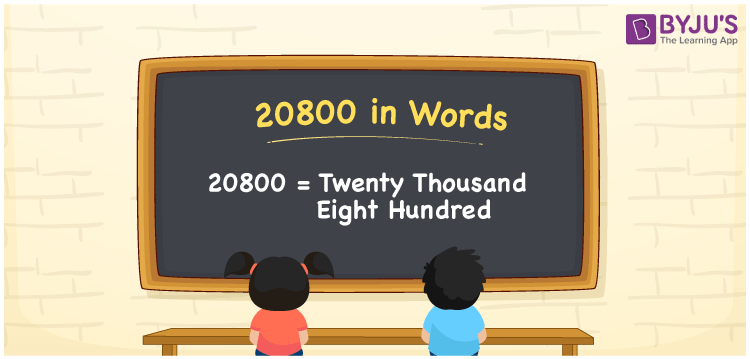# 20800 in Words

20800 in words can be written as Twenty Thousand Eight Hundred. The article of 20800 in words will provide students with a clear knowledge of the concept of counting numbers. If Rs. 20800 is spent on buying new chairs, then you can say that “I spent Twenty Thousand Eight Hundred Rupees in buying new chairs”. The  concept of converting 20800 into words using the English alphabet is discussed here. Students will be able to convert numbers in words with the help of the resources available at BYJU’S. 20800 can be read as “Twenty Thousand Eight Hundred” in English.

 20800 in words Twenty Thousand Eight Hundred Twenty Thousand Eight Hundred in Numbers 20800

## 20800 in English Words## How to Write 20800 in Words?

In this section you will learn in detail about conversion of 20800 into words using the place value chart. Five digits are present in 20800. The place value chart of 20800 can be understood easily using the table below.

 Ten Thousands Thousands Hundreds Tens Ones 2 0 8 0 0

The expanded form of the number 20800 is given below:

2 x Ten Thousand + 0 × Thousand + 8 × Hundred + 0 × Ten + 0 × One

= 2 x 10000 + 0 × 1000  + 8 × 100 + 0 × 10 + 0 × 1

= 20000 + 0000 + 800

= 20800

= Twenty Thousand Eight Hundred

Hence, 20800 in words is written as Twenty Thousand Eight Hundred.

20800 is a natural number that precedes 20801 and succeeds 20799.

20800 in words – Twenty Thousand Eight Hundred

Is 20800 an odd number? – No

Is 20800 an even number? – Yes

Is 20800 a perfect square number? – No

Is 20800 a perfect cube number? – No

Is 20800 a prime number? – No

Is 20800 a composite number? – Yes

## Frequently Asked Questions on 20800 in Words

### Write 20800 in words.

20800 can be written as “Twenty Thousand Eight Hundred” in words.

### Is 20800 an odd or even number?

20800 is an even number because it is divisible by 2.
20800/2 = 10400

### Write Twenty Thousand Eight Hundred in numbers.

Twenty Thousand Eight Hundred can be written in numbers as 20800.